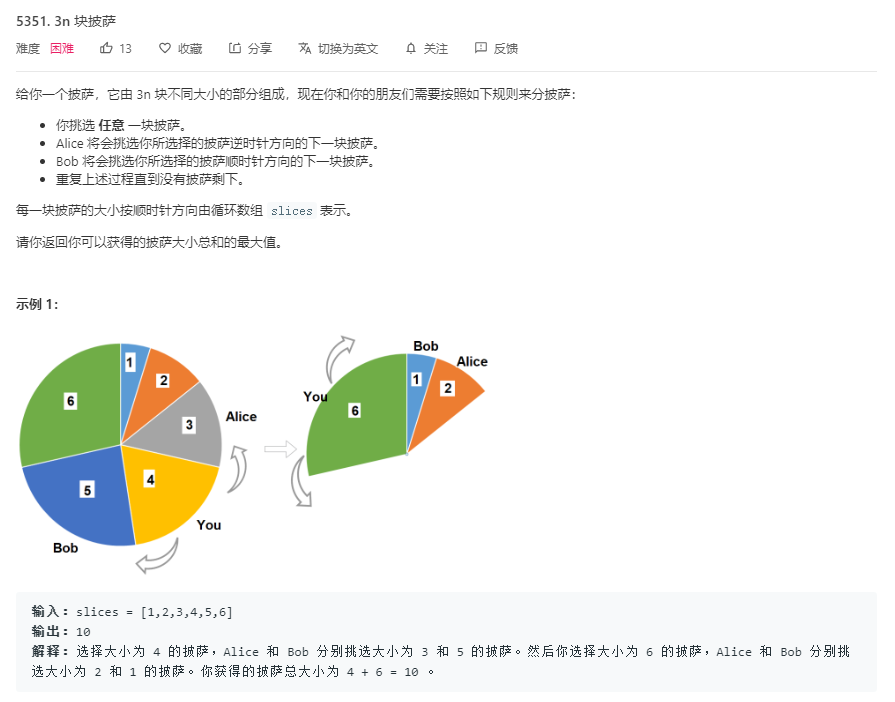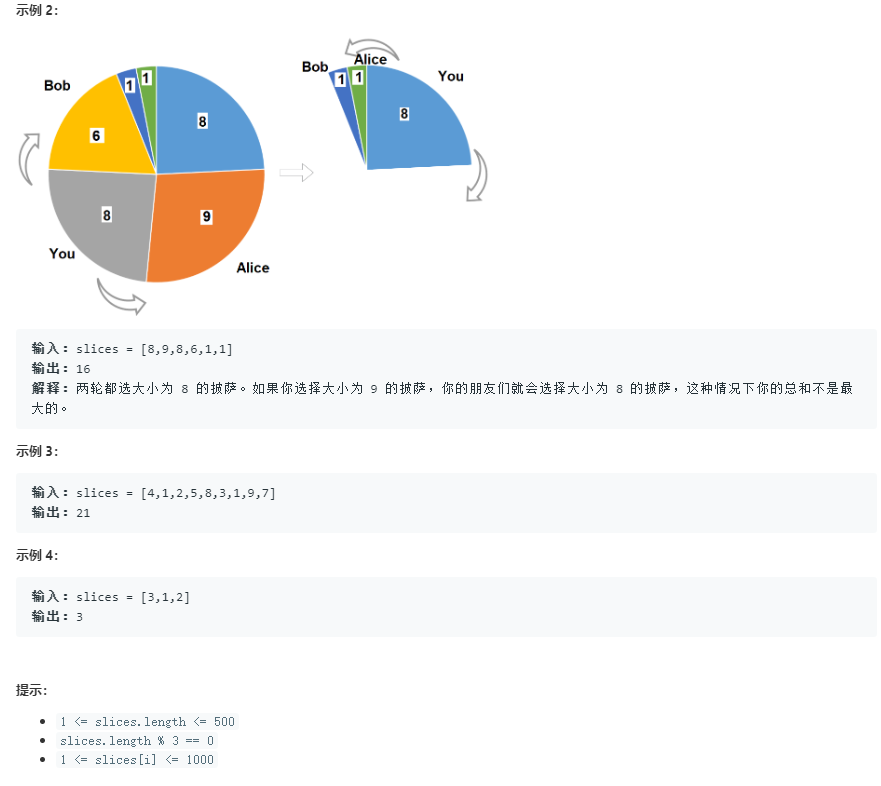## 2. 题目说明## 3. 题目解析

### 方法一：区间dp+环转区间技巧+巧妙解法

• 为什么使用 区间 `dp`？因为该问题具有区间性质？不是环吗，为什么成区间了？环可以变成区间…

• 首先分析其区间性质，在示例一中，我取 4 号披萨，`B` 取 5 号披萨， `A` 取 3 号披萨，可将其抽象化为一段连续的子区间。那么示例一再次进行取披萨时就不连续了，为什么不连续呢？那就是第一次取连续披萨所导致的。那么这个问题就与一段区间产生了关系。就尝试下 区间 `dp` 解决问题。

• 朴素区间 `dp` 设计，先不考虑环：

• 状态定义：

• `dp[l][r]`：取完区间 `l-->r` 的最大获取量
• 转移方程：

• 情况1 ：`dp[l1][r1]` 被取完，同时有 `dp[l2][r2]` 被取完，且有 `r1 + 1 = l2` 即这两个区间连续，那么就可以将区间进行合并为 `dp[l1][r2]` 即最大获取量等于两者的和。即找到两个相邻子区间的答案将其合并为一个大区间。
• 情况2： 假设当前在 `mid` 位置取到一个披萨，`A` 向左在 `l` 处取到一个披萨，`B` 向右在 `r` 处取到一个披萨。即 `l, mid, r` 那么就产生了 `dp[l][r]` 区间。那么产生这种情况有什么要求呢？必须满足两个严苛的条件，即 `l+1----mid - 1` 必须全部取完了这样 `A` 才会一直轮空取到第 `l` 块，同时 `mid + 1---r - 1` 必须也取完了，这样 `B` 才会一直轮空取到第 `r` 块。那么状态转移方程就可以表示为：`dp[i + 1][mid - 1] + slices[mid] + dp[mid + 1][r - 1]` 这三个的和用来更新 `dp[l][r]` 数组。这就是取披萨的操作
• 观察所以情况能够发现，取披萨操作即合并两相邻子区间操作就已经涵盖了所有的情况

• 如何断环？若不断环那么就会导致每次在数组边界时都得进行考虑，就会很麻烦。那么我们采用 区间 `dp` 的思想就需要将环处理成区间形式，这里直接采用一个技巧：倍增法，即将原数组 `1 2 3 4 5 6` 倍增为 `1 2 3 4 5 6 1 2 3 4 5 6` 这样就能将一个环的操作变成一个区间。再采用 区间 `dp` 操作即可

``````// 执行用时 :1252 ms, 在所有 C++ 提交中击败了100.00%的用户
// 内存消耗 :18.6 MB, 在所有 C++ 提交中击败了100.00%的用户

const int MAXN = 500 + 50;
int dp[MAXN * 3][MAXN * 3];

class Solution {
public:
int maxSizeSlices(vector<int>& slices) {
int n = slices.size();

memset(dp, -1, sizeof(dp));
for (int i = 0; i < n + n; i++)
dp[i][i + 2] = slices[(i + 1) % n];

for (int len = 3; len < n; len++){
if (len % 3 != 2) continue;
for (int left = 0; left < n + n; left++){
int right = left + len;
if (right >= n + n) continue;
for (int mid = left + 1; mid < right; mid++){
if ((mid - left - 1) % 3 != 0) continue;
if ((right - mid - 1) % 3 != 0) continue;
int cur = slices[mid % n];
if (mid != left + 1){
if (dp[left + 1][mid - 1] == -1) continue;
cur += dp[left + 1][mid - 1];
}
if (mid + 1 != right){
if (dp[mid + 1][right - 1] == -1) continue;
cur += dp[mid + 1][right - 1];
}
dp[left][right] = max(dp[left][right], cur);
}
for (int mid = left; mid < right; mid++){
if (dp[left][mid] == -1) continue;
if (dp[mid + 1][right] == -1) continue;
dp[left][right] = max(dp[left][right], dp[left][mid] + dp[mid + 1][right]);
}
}

}
int ans = -1;
for (int i = 0; i < n; i++) if (dp[i][i + n - 1] != -1) ans = max(ans, dp[i][i + n - 1]);

return ans;
}
};
``````